Test: Magnetostatics- 2

# Test: Magnetostatics- 2 - Electrical Engineering (EE)

Test Description

## 20 Questions MCQ Test Topicwise Question Bank for Electrical Engineering - Test: Magnetostatics- 2

Test: Magnetostatics- 2 for Electrical Engineering (EE) 2023 is part of Topicwise Question Bank for Electrical Engineering preparation. The Test: Magnetostatics- 2 questions and answers have been prepared according to the Electrical Engineering (EE) exam syllabus.The Test: Magnetostatics- 2 MCQs are made for Electrical Engineering (EE) 2023 Exam. Find important definitions, questions, notes, meanings, examples, exercises, MCQs and online tests for Test: Magnetostatics- 2 below.
Solutions of Test: Magnetostatics- 2 questions in English are available as part of our Topicwise Question Bank for Electrical Engineering for Electrical Engineering (EE) & Test: Magnetostatics- 2 solutions in Hindi for Topicwise Question Bank for Electrical Engineering course. Download more important topics, notes, lectures and mock test series for Electrical Engineering (EE) Exam by signing up for free. Attempt Test: Magnetostatics- 2 | 20 questions in 60 minutes | Mock test for Electrical Engineering (EE) preparation | Free important questions MCQ to study Topicwise Question Bank for Electrical Engineering for Electrical Engineering (EE) Exam | Download free PDF with solutions
 1 Crore+ students have signed up on EduRev. Have you?
Test: Magnetostatics- 2 - Question 1

### A circular conductor of 1 cm radius has an internal magnetic field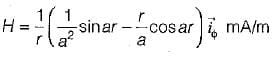where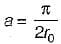, r0 is the radius of the conductor and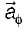is the unit vector. The total current in the conductor is given by

Detailed Solution for Test: Magnetostatics- 2 - Question 1

By Ampere's law,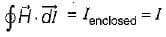Hence, current enclosed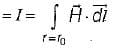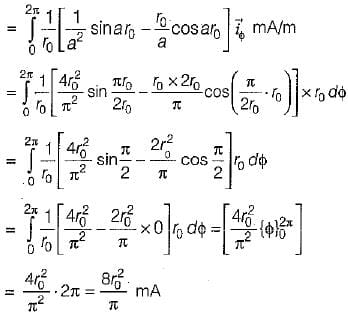Given, r0 = 1 cm = 0.01 m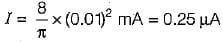Test: Magnetostatics- 2 - Question 2

### What is the value of mutual inductance between an infinitely long straight conductor along the y-axis and a rectangular single turn coil situated in x-y plane with its corners located at point (a, 0), (a + d, 0), (a, h) and (a + d, h) as shown in figure below?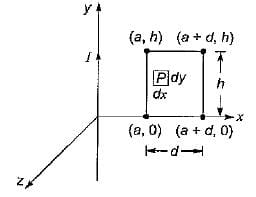Detailed Solution for Test: Magnetostatics- 2 - Question 2

The mutual inductance is given by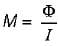Flux density at any point P in the x-y plane is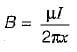and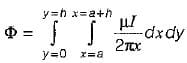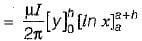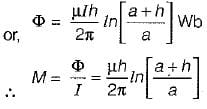Test: Magnetostatics- 2 - Question 3

### Assertion (A): The force acting between two parallel wires carrying currents is directly proportional to the individual currents and inversely proportional to the square of the distance between them, Reason (R): The force is repulsive if the two currents I1 and I2 are in the same directions and attractive if in opposite directions.

Detailed Solution for Test: Magnetostatics- 2 - Question 3

The force acting between the two parallel wires carrying currents I2 and I2 respectively is given by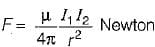Thus,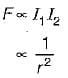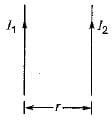Hence, assertion is a correct statement.
Reason is a false statement because F will be attractive if the two currents I2 and I2 will be in the same directions and repulsive if in opposite directions.

Test: Magnetostatics- 2 - Question 4

The energy stored in a magnetic field is given by

Test: Magnetostatics- 2 - Question 5

Lorentz force law is

Test: Magnetostatics- 2 - Question 6

The equation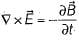is the generalization of

Test: Magnetostatics- 2 - Question 7

The unit of relative permeability is

Detailed Solution for Test: Magnetostatics- 2 - Question 7

Relative permeability,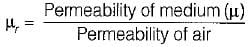μr is a dimensionless quantity.

Test: Magnetostatics- 2 - Question 8

Consider the volume current density distribution in cylindrical co-ordinates as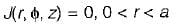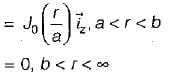Where a and b are inner and outer radii of the cylinder as shown in figure below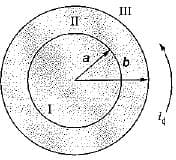Now, consider the value of magnetic field intensity in various regions I, II and III respectively obtained as: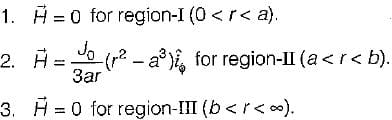Which of the above found values of H are correct for the respective regions?

Detailed Solution for Test: Magnetostatics- 2 - Question 8
• For region-I (0 < r < a), J (r, ϕ, z) = 0
Using Ampere’s law, we have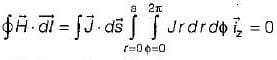or , H·2πr = 0 or H = 0
• For region - II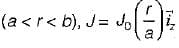Again,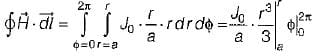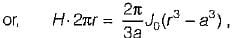or,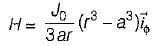• For region - III  (b < r < ∞), J = 0
Again,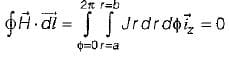Test: Magnetostatics- 2 - Question 9

Assertion (A): It is not possible to have isolated magnetic charges.
Reason (R): The magnetic flux lines always close upon themselves.

Detailed Solution for Test: Magnetostatics- 2 - Question 9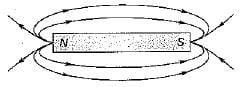Since, magnetic flux lines always close upon themselves therefore it is not possible to have isolated magnetic charges (or poles).

Test: Magnetostatics- 2 - Question 10

Match List-l with List-lI and select the correct answer using the codes given below the fists:
List-l
A. Gauss’s law for magnetostatic fields
B. Gauss’s law for electrostatic fields
C. Conservativeness of electrostatic fields
D. Ampere's law
List-lI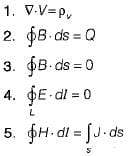Codes:
A B C D
(a) 5 4 1 2
(b) 3 1 4 5
(c) 3 4 1 5
(d) 5 1 4 2

Test: Magnetostatics- 2 - Question 11

The magnetic vector potential is given by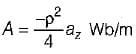The total magnetic flux crossing the surface ϕ = π/2 , 1 ≤ ρ ≤ 2 m , 0 ≤ z ≤ 5 m is

Detailed Solution for Test: Magnetostatics- 2 - Question 11

The total magnetic flux crossing the surface
ϕ = π/2 , 1 ≤ ρ ≤ 2 m , 0 ≤ z ≤ 5 m is given as: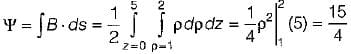= 3.75 Wb

Test: Magnetostatics- 2 - Question 12

Which of the following is not a source of magnetostatic fields?

Detailed Solution for Test: Magnetostatics- 2 - Question 12

An accelerated charge will produce both time varying electric and magnetic field called electromagnetic field.

Test: Magnetostatics- 2 - Question 13

Consider the following statements associated with the characteristics of static magnetic field:
1. It is solenoidal.
2. Magnetic flux lines are not closed in certain cases.
3. The total number of flux lines entering a given region is equal to the total number of flux lines leaving the region.
4. It is conservative.
Which of the above statements is/are not correct?

Detailed Solution for Test: Magnetostatics- 2 - Question 13
• Static magnetic field is non-conservative unlike static electric field. Hence, statement-4 is not correct.
• Magnetic flux lines are always closed. Hence, statement-2 is not correct.
• Statement 1 and 3 are correct.
Test: Magnetostatics- 2 - Question 14

What are the units for the product of two values whose respective units are Henrys and Amperes?

Detailed Solution for Test: Magnetostatics- 2 - Question 14

The Henry, the unit for mutual inductance is equivalent to V-sec/Ampere.
So, H x Ampere = Volt-sec = V-s

Test: Magnetostatics- 2 - Question 15

What are the possible dimensions for a rectangular coil that has a magnetic flux of 9.5 webers when in a magnetic field of strength 19 Tesla at an angle of 60° from the perpendicular to the plane of the coil?

Detailed Solution for Test: Magnetostatics- 2 - Question 15

Magnetic flux φB = BA cosθ
or,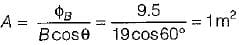Hence, the area of the rectangular coil should be 1 m2. Only option (d) is matching the answer i.e. 250 cm x 40 cm
= 10000 cm2 = 1 m2.

Test: Magnetostatics- 2 - Question 16

What is the change in magnetic fiux in a coil of area 5 m2 as its orientation relative to the perpendicular of a uniform 3.0 T magnetic field changes from 45° to 90°?

Detailed Solution for Test: Magnetostatics- 2 - Question 16

Flux ϕ = B A cosθ
At θ = 45°,   ϕ1= 3 x 5 x cos 45° = 11 Wb
At θ = 90°,    ϕ2 = 3 x 5 x cos 90° = 0 Wb
∴ Change in flux = ϕ2 - ϕ1 = -11 Wb

Test: Magnetostatics- 2 - Question 17

If a vector field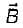is solenoidal, which of these is true?

Detailed Solution for Test: Magnetostatics- 2 - Question 17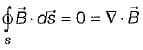(Non-existence of monopole)

Test: Magnetostatics- 2 - Question 18

If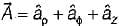, the value of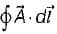around the closed circular quadrant shown in the given figure is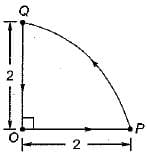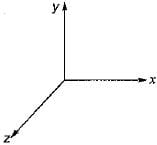Detailed Solution for Test: Magnetostatics- 2 - Question 18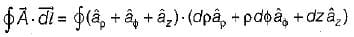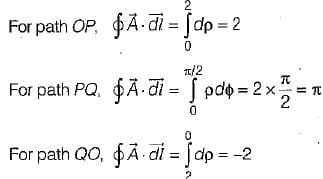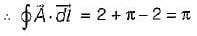Test: Magnetostatics- 2 - Question 19

The Maxwell’s equation,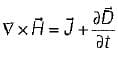is based on

Test: Magnetostatics- 2 - Question 20

Given a vector field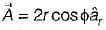in cylindrical coordinates. For the contour as shown below,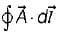is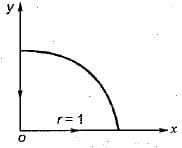Detailed Solution for Test: Magnetostatics- 2 - Question 20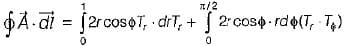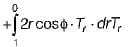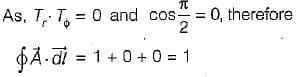## Topicwise Question Bank for Electrical Engineering

211 tests
Information about Test: Magnetostatics- 2 Page
In this test you can find the Exam questions for Test: Magnetostatics- 2 solved & explained in the simplest way possible. Besides giving Questions and answers for Test: Magnetostatics- 2, EduRev gives you an ample number of Online tests for practice

211 tests